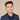# How To Check If An Element Is In An Array In Swift

Swift makes it easy to check if an element is in an array

## Use The Contains Method

The contains method in Swift is the best way to check if an element is in an array:

``````let myArray = [1, 2, 3]
if myArray.contains(3) {
print("found element")
}``````

The contain method returns a boolean.

Note that this requires the elements of the array conform to the `Equatable` protocol.

## How To Remove Specific Element In Array

Sometimes you want to check if an array element exists, and if it does, you want to remove it.

``````var myArray = [1, 2, 3]
if let index = myArray.firstIndex(of: 3) {
myArray.remove(at: index)
}``````

The index function returns an integer of where the object is in the array.

Note that the remove function only removes the first element of the array.

## Get All Instances Of A Specific Object In An Array

``````let myArray = [1, 2, 3, 3, 3]
let results = myArray.filter { \$0 == 3 }
print(results)``````

This prints:

``[3, 3, 3]``

Note we could check if this results array is empty to see if there are any elements:

``````if results.isEmpty {
} else {
print("found!")
}``````

## Get The Count Of A Certain Element In An Array

To get the count, simply add a `.count` to the end of our code.

``````let myArray = [1, 2, 3, 3, 3]
let results = myArray.filter { \$0 == 3 }
print(results.count)``````

This results in

``3``

## Remove All Instances Of A Specific Object In An Array

Use the filter and set your condition.

``````let myArray = [1, 2, 3, 3, 3]
let results = myArray.filter { \$0 != 3 }
print(results)``````

Here, we set our condition to `!= 3` so the results array will only contain elements that are not 3.

The above code prints:

``[1, 2]``

## Get All Objects That Match A Property

Say we have a custom class named `MyClass`. We can get all elements that match a certain property by using filter again.

``````class MyClass {
var someProperty: Int

init(someProperty: Int) {
self.someProperty = someProperty
}
}

let myClassArray: [MyClass] = [MyClass(someProperty: 2), MyClass(someProperty: 3)]

let results = myClassArray.filter {\$0.someProperty == 2}

print(results.someProperty)
print(results.count)``````

This prints:

``````2
1``````
If you liked this post and want to learn more, check out The Complete iOS Developer Bootcamp. Speed up your learning curve - hundreds of students have already joined. Thanks for reading!## Eddy Chung

I teach iOS development on ZeroToAppStore.com.